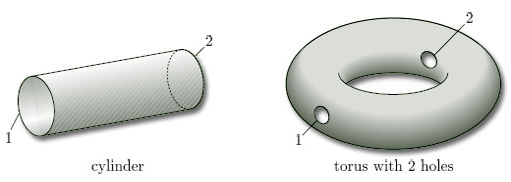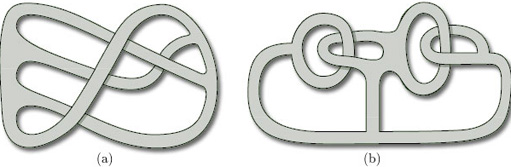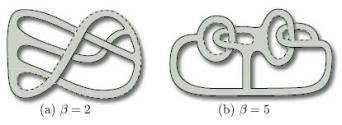Surfaces

Start this free course now. Just create an account and sign in. Enrol and complete the course for a free statement of participation or digital badge if available.

Free course

# 2.2.4 Boundary numbers

In a surface with boundary, the boundary may consist of more than one piece. For example, the boundary of a cylinder, or of a torus with two holes, consists of two pieces, as shown in Figure 23.Figure 23

The separate pieces forming the boundary of a surface S are called the boundary components of S. The number of boundary components is the boundary number of S, denoted by β. Thus β = 2 for each of the surfaces in Figure 23.

For a surface without boundary, there are no boundary components, so β = 0.

(β is the Greek letter beta.)

## Problem 7

For each of the surfaces in Figure 24, write down the boundary number β.

(a) β = 1; (b) β = 4; (c) β = 1

To find the boundary numbers of paper surfaces, it is convenient to use coloured pens to trace around the boundary. Doing this, we find that the two boundary numbers of the surfaces in Figure 22 are β = 4 and β = 3, as shown in Figure 25.

## Problem 8

Find the boundary number β of each of the paper surfaces in Figure 26.Figure 26The boundary number β is one of the three characteristic numbers that we use to classify compact surfaces.

M338_1